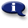# .

## Syllabus Information

Fall 2022
May 31,2023Use this page to maintain syllabus information, learning objectives, required materials, and technical requirements for the course.

Syllabus Information
MTH 020D - Math Renewal: Part D
Associated Term: Fall 2022
Learning Objectives: Upon successful completion of this course, the student will: 1- Apply reasoning and problem solving skills to basic mathematics problems. 2- Check the reasonableness of answers. Solve applications using area and perimeter of simple geometric shapes. 3- Solve basic equations of the type a + x = b and ax = b, involving whole numbers, fractions and decimals. 4- Use a scientific calculator to explore and solve basic math problems. 5- Apply study skills for learning mathematics and for coping with math anxiety. 6- Use the symbols and vocabulary of basic mathematics correctly. Use American and metric measurement.
Required Materials:
Technical Requirements: